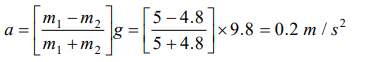## Laws of Motion Questions and Answers Part-19

1.Three blocks of masses $m_{1},m_{2}$  and $m_{3}$ are connected by massless strings as shown on a frictionless table. They are pulled with a force $T_{3}=40N$  . If $m_{1}=10kg$   , $m_{2}=6kg$  and $m_{3}=4kg$  , the tension $T_{2}$ will bea) 20 N
b) 40 N
c) 10 N
d) 32 N

Explanation: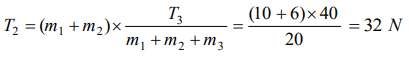2. A block of mass $m_{1}$ rests on a horizontal table. A string tied to the block is passed on a frictionless pulley fixed at the end of the table and to the other end of string is hung another block of mass $m_{2}$ . The acceleration of the system is
a) $\frac{m_{2}g}{\left(m_{1}+m_{2}\right)}$
b) $\frac{m_{1}g}{\left(m_{1}+m_{2}\right)}$
c) g
d) $\frac{m_{2}g}{m_{1}}$

Explanation: $\frac{m_{2}g}{\left(m_{1}+m_{2}\right)}$

3. A 2 kg block is lying on a smooth table which is connected by a body of mass 1 kg by a string which passes through a pulley. The 1 kg mass is hanging vertically. The acceleration of block and tension in the string will be
a) $3.27m\diagup s^{2},6.54 N$
b) $4.38m\diagup s^{2},6.54 N$
c) $3.27m\diagup s^{2},9.86 N$
d) $4.38m\diagup s^{2},9.86 N$

Explanation:4. A light string passes over a frictionless pulley. To one of its ends a mass of 6 kg is attached. To its other end a mass of 10 kg is attached. The tension in the thread will bea) 24.5 N
b) 2.45 N
c) 79 N
d) 73.5 N

Explanation: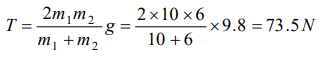5. Two masses of 5kg and 10kg are connected to a pulley as shown. What will be the acceleration of the system (g= acceleration due to gravity)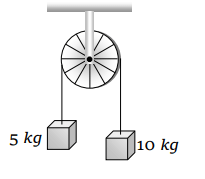a) g
b) $\frac{g}{2}$
c) $\frac{g}{3}$
d) $\frac{g}{4}$

Explanation:6. A block A of mass 7 kg is placed on a frictionless table. A thread tied to it passes over a frictionless pulley and carries a body B of mass 3 kg at the other end. The acceleration of the system is $\left(g=10m s^{-2}\right)$a) $100m s^{-2}$
b) $3m s^{-2}$
c) $10m s^{-2}$
d) $30m s^{-2}$

Explanation: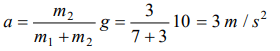7. Three blocks of masses 2 kg, 3 kg and 5 kg are connected to each other with light string and are then placed on a frictionless surface as shown in the figure. The system is pulled by a force $F=10N$  , then tension $T_{1}=$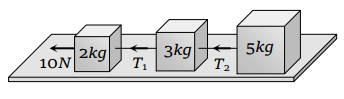a) 1N
b) 5 N
c) 8 N
d) 10 N

Explanation:8. A light string passing over a smooth light pulley connects two blocks of masses m1 and m2 (vertically). If the acceleration of the system is g/8 then the ratio of the masses is
a) 8 : 1
b) 9 : 7
c) 4 : 3
d) 5 : 3

Explanation:9. Two masses $m_{1}=5 kg$  and $m_{2}=4.8kg$   tied to a string are hanging over a light frictionless pulley. What is the acceleration of the masses when they are free to move $\left(g=9.8m\diagup s^{2}\right)$a) $0.2m\diagup s^{2}$
b) $9.8 m\diagup s^{2}$
c) $5m\diagup s^{2}$
d) $4.8m\diagup s^{2}$